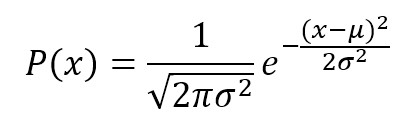/  Top Machine learning interview questions and answers   /  What is the difference between Gaussian, Multinomial and Bernoulli Naïve Bayes classifiers?## What is the difference between Gaussian, Multinomial and Bernoulli Naïve Bayes classifiers?

### Gaussian Naive Bayes

Gaussian Naive Bayes is useful when working with continuous values which probabilities can be modeled using a Gaussian distribution:

### Multinomial naive Bayes

A multinomial distribution is useful to model feature vectors where each value represents, for example, the number of occurrences of a term or its relative frequency. If the feature vectors have elements and each of them can assume different values with probability pk, then:

### Bernoulli naive Bayes

If X is random variable Bernoulli-distributed, it can assume only two values (for simplicity, let’s call them 0 and 1) and their probability is: